#### A zero-dimensional variety

Now a kinematic closure constraint will be imposed. Fix the point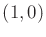in the body frame of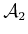at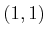in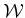. This yields the constraints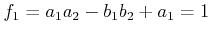(4.62)

and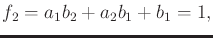(4.63)

by substituting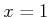and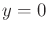into (4.60) and (4.61). This yields the variety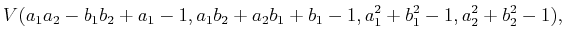(4.64)

which is a subset of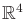. The polynomials are slightly modified because each constraint must be written in the form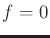.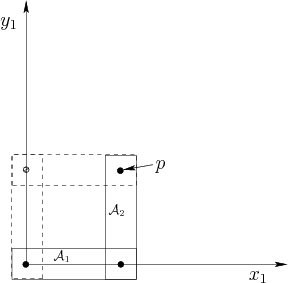Although (4.64) represents the constrained configuration space for the chain of two links, it is not very explicit. Without an explicit characterization (i.e., a parameterization), it complicates motion planning. From Figure 4.22 it can be seen that there are only two solutions. These occur for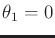,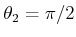and,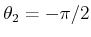. In terms of the polynomial variables,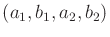, the two solutions are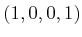and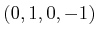. These may be substituted into each polynomial in (4.64) to verify that 0 is obtained. Thus, the variety represents two points in. This can also be interpreted as two points on the torus,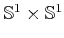.

It might not be surprising that the set of solutions has dimension zero because there are four independent constraints, shown in (4.64), and four variables. Depending on the choices, the variety may be empty. For example, it is physically impossible to bring the point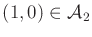to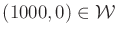.

Steven M LaValle 2020-08-14